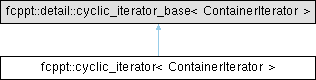4.4.0 Freundlich's C++ toolkit
Searching...
No Matches
fcppt::cyclic_iterator< ContainerIterator > Class Template Referencefinal

## Detailed Description

template<typename ContainerIterator>
class fcppt::cyclic_iterator< ContainerIterator >

An iterator adaptor that cycles through a range.

Template Parameters
 ContainerIterator The underlying iterator which must be at least a forward iterator

A cyclic iterator can be useful in cases where you want `end()` to become `begin()` again. For example, imagine a cycling through a list of items which means if you skip over the last, you will return to the first one.

Here is a short example demonstrating its use.

using int_vector = std::vector<int>;
int_vector const ints{{1, 2}};
const_cyclic_iterator it(
ints.begin(), // start position of the iterator
const_cyclic_iterator::boundary{
ints.begin(), // start of the container
ints.end() // end of the container
});
// prints 1, 2, 1, 2, 1, 2,
fcppt::algorithm::repeat(6, [&it] { std::cout << *it++ << ", "; });
std::cout << '\n';
Inheritance diagram for fcppt::cyclic_iterator< ContainerIterator >:## Public Types

using base_type = fcppt::detail::cyclic_iterator_base< ContainerIterator >

using container_iterator_type = ContainerIterator

using value_type = typename base_type::value_type

using reference = typename base_type::reference

using pointer = typename base_type::pointer

using difference_type = typename base_type::difference_type

using iterator_category = typename base_type::iterator_category

using boundary = fcppt::tuple::object< container_iterator_type, container_iterator_type >

## Public Member Functions

cyclic_iterator ()

template<typename OtherIterator >
cyclic_iterator (cyclic_iterator< OtherIterator > const &other)
Copy constructs from another cyclic iterator.

cyclic_iterator (container_iterator_type const &pos, boundary)
Constructs a new cyclic iterator.

template<typename OtherIterator >
cyclic_iteratoroperator= (cyclic_iterator< OtherIterator > const &other)
Assigns from another cyclic iterator.

boundary const & get_boundary () const
Returns the boundary.

container_iterator_type get () const
Returns the underlying iterator.

void increment ()

void decrement ()

bool equal (cyclic_iterator const &) const

reference dereference () const

difference_type distance_to (cyclic_iterator const &) const

## ◆ base_type

template<typename ContainerIterator >
 using fcppt::cyclic_iterator< ContainerIterator >::base_type = fcppt::detail::cyclic_iterator_base

## ◆ boundary

template<typename ContainerIterator >
 using fcppt::cyclic_iterator< ContainerIterator >::boundary = fcppt::tuple::object

## ◆ container_iterator_type

template<typename ContainerIterator >
 using fcppt::cyclic_iterator< ContainerIterator >::container_iterator_type = ContainerIterator

## ◆ difference_type

template<typename ContainerIterator >
 using fcppt::cyclic_iterator< ContainerIterator >::difference_type = typename base_type::difference_type

## ◆ iterator_category

template<typename ContainerIterator >
 using fcppt::cyclic_iterator< ContainerIterator >::iterator_category = typename base_type::iterator_category

## ◆ pointer

template<typename ContainerIterator >
 using fcppt::cyclic_iterator< ContainerIterator >::pointer = typename base_type::pointer

## ◆ reference

template<typename ContainerIterator >
 using fcppt::cyclic_iterator< ContainerIterator >::reference = typename base_type::reference

## ◆ value_type

template<typename ContainerIterator >
 using fcppt::cyclic_iterator< ContainerIterator >::value_type = typename base_type::value_type

## ◆ cyclic_iterator() [1/3]

template<typename ContainerIterator >
 fcppt::cyclic_iterator< ContainerIterator >::cyclic_iterator ( )

## ◆ cyclic_iterator() [2/3]

template<typename ContainerIterator >
template<typename OtherIterator >
 fcppt::cyclic_iterator< ContainerIterator >::cyclic_iterator ( cyclic_iterator< OtherIterator > const & other )
explicit

Copy constructs from another cyclic iterator.

Copy constructs from another cyclic iterator other. This only works if the underlying iterators are convertible.

Parameters
 other The iterator to copy construct from

## ◆ cyclic_iterator() [3/3]

template<typename ContainerIterator >
 fcppt::cyclic_iterator< ContainerIterator >::cyclic_iterator ( container_iterator_type const & pos, boundary )

Constructs a new cyclic iterator.

Constructs a new cyclic iterator, starting at it, inside a range of boundary.

## Member Function Documentation

template<typename ContainerIterator >
 void fcppt::cyclic_iterator< ContainerIterator >::advance ( difference_type )

## ◆ decrement()

template<typename ContainerIterator >
 void fcppt::cyclic_iterator< ContainerIterator >::decrement ( )

## ◆ dereference()

template<typename ContainerIterator >
 reference fcppt::cyclic_iterator< ContainerIterator >::dereference ( ) const

## ◆ distance_to()

template<typename ContainerIterator >
 difference_type fcppt::cyclic_iterator< ContainerIterator >::distance_to ( cyclic_iterator< ContainerIterator > const & ) const

## ◆ equal()

template<typename ContainerIterator >
 bool fcppt::cyclic_iterator< ContainerIterator >::equal ( cyclic_iterator< ContainerIterator > const & ) const

## ◆ get()

template<typename ContainerIterator >
 container_iterator_type fcppt::cyclic_iterator< ContainerIterator >::get ( ) const

Returns the underlying iterator.

## ◆ get_boundary()

template<typename ContainerIterator >
 boundary const & fcppt::cyclic_iterator< ContainerIterator >::get_boundary ( ) const

Returns the boundary.

## ◆ increment()

template<typename ContainerIterator >
 void fcppt::cyclic_iterator< ContainerIterator >::increment ( )

## ◆ operator=()

template<typename ContainerIterator >
template<typename OtherIterator >
 cyclic_iterator & fcppt::cyclic_iterator< ContainerIterator >::operator= ( cyclic_iterator< OtherIterator > const & other )

Assigns from another cyclic iterator.

Assigns from another cyclic iterator other. This only works if the underlying iterators are convertible.

Parameters
 other The iterator to assign from
Returns
`*this`